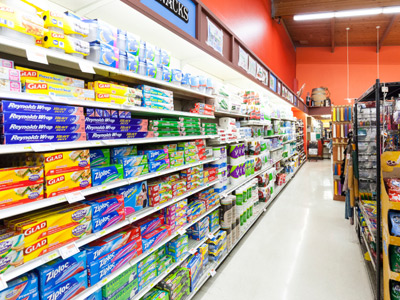Knowing percentages really helps when shopping!

# Calculating Percentages

This Math quiz is called 'Calculating Percentages' and it has been written by teachers to help you if you are studying the subject at middle school. Playing educational quizzes is a fabulous way to learn if you are in the 6th, 7th or 8th grade - aged 11 to 14.

It costs only \$12.50 per month to play this quiz and over 3,500 others that help you with your school work. You can subscribe on the page at Join Us

By now you may feel that you are pretty comfortable with percentages but it is always an important asset to refresh what you know. This goes along with the phrase, “practice makes perfect.” So in this quiz we are going to revisit how to calculate percentages.

Do you remember what a percentage is? A percentage is a way in which you can express a fraction (or a part of a whole) in portions of 100. In word form, a percentage would look like this:

Part/Whole = Percent/100

Instead of using the word “percent” we use the symbol “%”.

Here is a quick refresher on how to find the percentage of any given number. If you were to go to the grocery store and a sign showed that a product is 25% off, you would need to know what the price is of the product that is being discounted by 25%. Let’s say the product is laundry detergent and it normally sells for \$8.00. You now know you need to find out what is 25% of \$8.00. To do this you must multiply the amount of \$8.00 by the percent “25”. However if you multiply 8.00 x 25, you would get the answer: “200.00”. That certainly cannot be right. Remember that a percentage needs to be converted to a decimal point when you multiply. 25% = .25 as it is 25 of 100 (i.e., 25/100). So we need to multiply \$8.00 x .25 which then equals \$2.00. \$8.00 - \$2.00 = \$6.00. You can buy the laundry detergent for \$6.00.

For each percentage problem listed below, locate the answer that will show the correct number and/or percentage. (Round numbers to the nearest 100th.)

1.
17% of 13 =
221
22.1
2.21
.22
Remember to convert the percentage to a decimal point so the problem will now look like this: 13 x .17 = 2.21. Answer (c) is the correct answer
2.
57% of 319 =
181.83
18.18
1818.3
1.82
Remember to convert the percentage to a decimal point so the problem will now look like this: 319 x .57 = 181.83. Answer (a) is the correct answer
3.
12% of 94 =
112.8
1.13
11.28
113
Remember to convert the percentage to a decimal point so the problem will now look like this: 94 x .12 = 11.28. Answer (c) is the correct answer
4.
36% of 824 =
29.7
297
29.6
296.64
Remember to convert the percentage to a decimal point so the problem will now look like this: 824 x .36 = 296.64. Answer (d) is the correct answer
5.
42% of 515 =
21.63
2.16
216.3
2163
Remember to convert the percentage to a decimal point so the problem will now look like this: 515 x .42 = 216.3. Answer (c) is the correct answer
6.
24% of 164 =
39.36
3.94
393.6
3936
Remember to convert the percentage to a decimal point so the problem will now look like this: 164 x .24 = 39.36. Answer (a) is the correct answer
7.
65% of 150 =
98
97.5
975
.98
Remember to convert the percentage to a decimal point so the problem will now look like this: 150 x .65 = 97.5. Answer (b) is the correct answer
8.
62% of 650 =
40.3
4.03
43
403
Remember to convert the percentage to a decimal point so the problem will now look like this: 650 x .62 = 403. Answer (d) is the correct answer
9.
22% of 89 =
19.58
195.8
.20
1.96
Remember to convert the percentage to a decimal point so the problem will now look like this: 89 x .22 = 19.58. Answer (a) is the correct answer
10.
28% of 7 =
2
1.96
19.6
196
Remember to convert the percentage to a decimal point so the problem will now look like this: 7 x .28 = 1.96. Answer (b) is the correct answer
Author:  Christine G. Broome